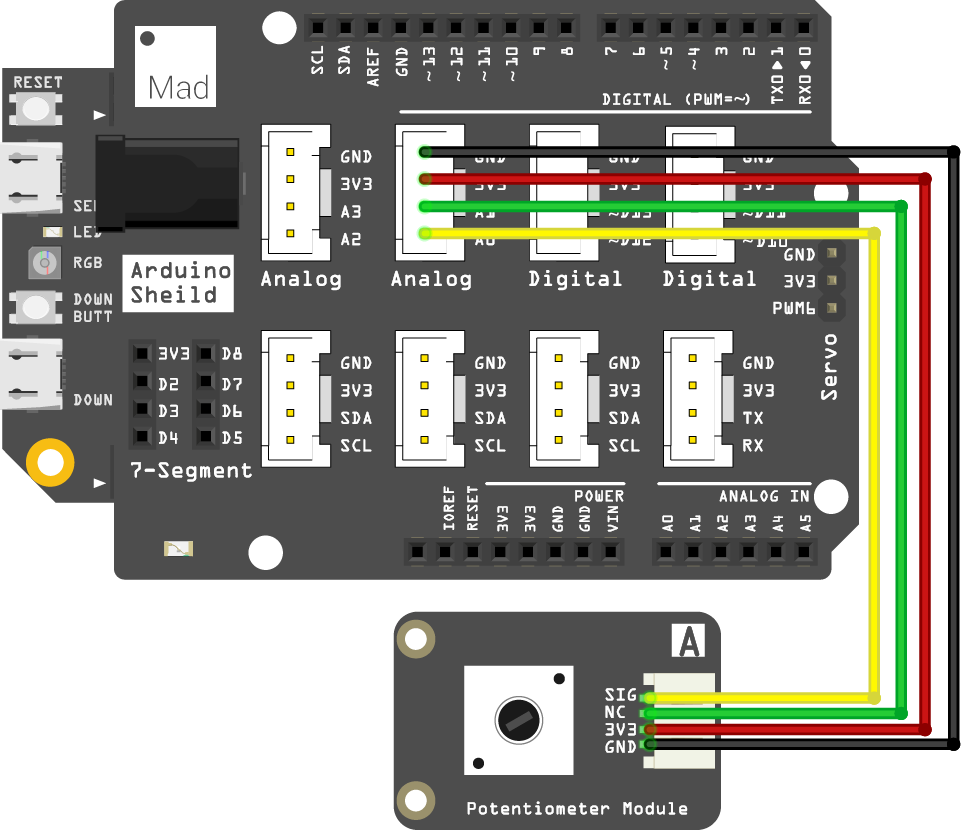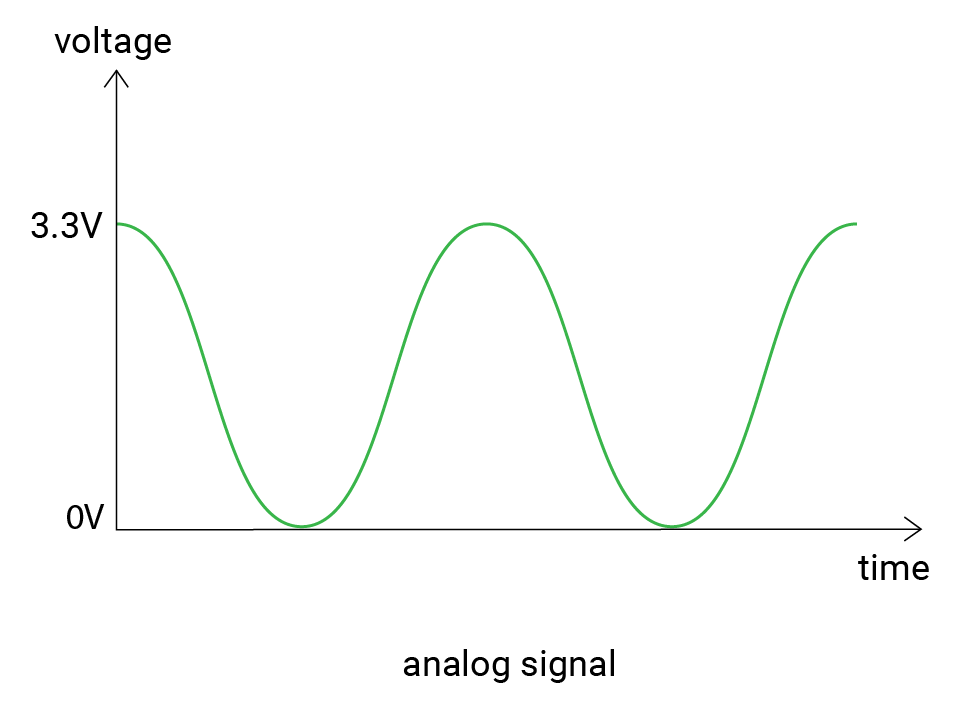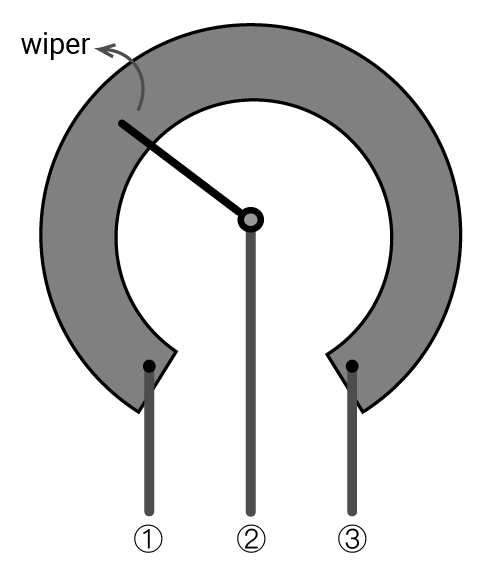# Mission4_Potentiometer_RGB

In this project, you'll turn a potentiometer to control the LED blink rate, to make it blink faster or slower. The potentiometer is widely used to control other devices. And you will often use it in the following projects.

## What you need​

The parts you will need are all included in the Maker kit.

• SwiftIO board
• Shield
• Potentiometer module
• 4-pin cable

## Circuit​

1. Place the shield on top of your SwiftIO board.

2. Connect the potentiometer module to pin A0 using a 4-pin cable.## Example code​

``// Import the SwiftIO library to use everything in it.import SwiftIO// Import the board library to use the Id of the specific board.import MadBoard// Import the SwiftIO library to use everything in it.let a0 = AnalogIn(Id.A0) // Initialize the red onboard led.let led = DigitalOut(Id.RED) while true {    // Alternate state of the LED.    led.toggle()    // Return the percentage of the voltage in the range of 0.0 to 1.0.    let analogValue = a0.readPercent()        // Stop the program for a certain period based on the value to keep current led state.    let delayTime = Int(analogValue * 500)    sleep(ms: delayTime)}``

## Background​

### Analog signal​

You have known that a digital signal has determined values. An analog signal is quite different. Its voltage changes smoothly with time. And its value ranges between 0V and 3.3V. So you can get 1.5V, 2V, 2.75V... There can be infinite possible values.So how does the board read the values? Here comes the analog to digital converter. In brief, it converts the analog voltage to a digital value that the microcontroller can read.

The converters have different precisions, so the resolution describes the possible values they can measure. Our boards have a 12-bit resolution, which means there can be 4096 (0-4095) values in total. The values from 0 to 4095 are known as raw values.

Then let's see the working process in detail. When the board reads from the analog pin, it will first get a raw value, which will be mapped to voltage value proportionally. Here is the formula:

resolution / reference voltage = raw value / actual voltage

where

resolution: 4096; reference voltage: 3.3V

For example, if the raw value equals 0, the voltage would be 0V; if the raw value equals 4095, the voltage would be 3.3V; and 2047 corresponds 1.65V.

And you can know from the formula that the resolution will influence the precision of the final result. A higher resolution gives a result with higher accuracy.

### Potentiometer​

The potentiometer is one kind of variable resistor. You can adjust its resistance by rotating its shaft clockwise or anticlockwise. The wiper inside it will change its position accordingly.As shown above, the resistance between ① and ③ is its maximum value. The wiper divides it into two parts. As the wiper moves, the resistances of the two parts will change.

## Code analysis​

``import SwiftIOimport MadBoard``

First, import the two libraries: SwiftIO and SwiftIOBoard. SwiftIO is used to control the input and output of the SwiftIO board. SwiftIOBoard defines the pin name of the board.

``let a0 = AnalogIn(Id.A0)let led = DigitalOut(Id.RED)``

Initialize the red onboard LED and the analog pin (A0) the potentiometer connects.

``led.toggle()``

`toggle()` is used to reverse the digital output voltage. If the voltage is high, it will be changed to low then, and vice versa. `led.toggle()` will change the state of the onboard LED automatically. You don't need to know if the LED is on or off.

``let analogValue = a0.readPercent()``

`a0.readPercent()` allows you to get the input voltage in percentage. It represents the ratio between the raw value and the resolution (4096).

``let delayTime = Int(analogValue * 500)sleep(ms: delayTime)``

The blink rate is decided by sleep time. Then this time is related to the input value. In this way, the potentiometer can control the LED.

## Reference​

DigitalOut - set whether the pin output a high or low voltage.